In June 2021, Ali algorithmic post had 6 interview questions
Seven children online in July 2021-08-09 18:57:56

This is my participation 8 The fourth of the yuegengwen challenge 2 God , Check out the activity details ：8 Yuegengwen challenge

## 1. Common loss function , Common activation functions ,ELU function Understand? ？

Common loss function ：0-1 Loss function , Absolute loss function ,log Logarithmic loss function , Square loss function , Exponential loss function ,hinge Loss function , Cross entropy loss function, etc .

0-1 Loss function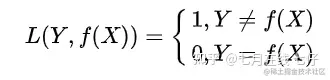Absolute loss function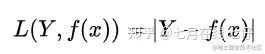log Logarithmic loss function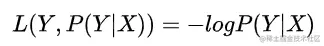Square loss function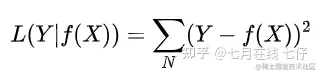Exponential loss function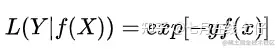hinge Loss function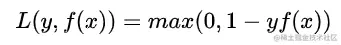Cross entropy loss function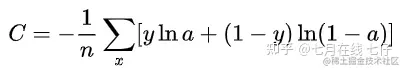Common activation functions are ：Sigmoid、Tanh、ReLU、Leaky ReLU

Sigmoid function ：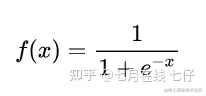characteristic ：

It can transform the continuous real value of the input into 0 and 1 Between the output , Special , If it's a very large negative number , So the output is 0; If it's a very large positive number , Output is 1.

shortcoming ：

shortcoming 1： In the depth neural network, the gradient disappears when the gradient is transferred back , The probability of gradient explosion is very small , The probability of gradient disappearance is relatively large .

shortcoming 2：Sigmoid Of output No 0 mean value （ namely zero-centered）.

shortcoming 3： Its analytical formula contains power operation , It's relatively time-consuming to solve by computer . For large-scale deep networks , This will greatly increase the training time .

Tanh function ：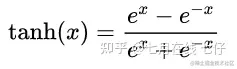characteristic ： It solves Sigmoid Function is not zero-centered Output problems , Convergence rate ratio sigmoid Be quick , However , The gradient disappears （gradient vanishing） And the problem of power operation still exists .

ReLU function ：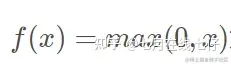characteristic ：

1.ReLu The function uses the threshold to output the dependent variable , Therefore, the computational complexity will be lower than the other two functions （ The last two functions are exponential operations ）

2.ReLu The non saturation of function can effectively solve the problem of gradient disappearance , Provides a relatively wide activation boundary .

3.ReLU The unilateral suppression provides the sparse expression ability of the network .

ReLU The limitations of : The problem is that it will lead to neuron death during training .

This is due to the function f(x)=max(0,x) Causes a negative gradient after passing through the ReLU Unit is set to 0, And will not be activated by any data later , That is, the gradient flowing through the neuron is always 0, No response to any data . In actual training , If learning rate （Learning Rate） Set large , It will cause more than a certain proportion of neurons to die irreversibly , Furthermore, the parameter gradient cannot be updated , The whole training process failed .

Leaky ReLu function ：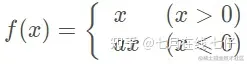LReLU And ReLU The difference is that , When z<0 Its value is not 0, It's a slope of a The linear function of , commonly a For a small normal number , In this way, unilateral inhibition is realized , Some negative gradient information is retained so that it is not completely lost . But on the other hand ,a The choice of value increases the difficulty of the problem , Strong artificial prior or repeated training is needed to determine the appropriate parameter value .

Based on this , A parameterized PReLU（Parametric ReLU） emerge as the times require . It is associated with LReLU The main difference is that the slope of the negative axis part a As a learnable parameter in the network , Back propagation training , Joint optimization with other network layers with parameters . And another one. LReLU Variants of have increased “ randomization ” Mechanism , In particular , In the process of training , Slope a As a random sample satisfying a certain distribution ; Then fix it during the test .Random ReLU（RReLU） To some extent, it can play the role of regularization .

ELU function ：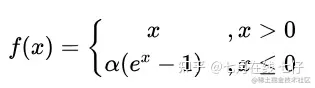ELU The function is for ReLU An improved form of function , Compared with ReLU function , When the input is negative , There is a certain output , And this part of the output also has a certain anti-interference ability . This can eliminate ReLU Dead questions , But there are still problems with gradient saturation and exponential operations .

## 2. Why not MSE, Instead, we use cross entropy .

LR The basic expression of is as follows ：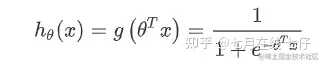The derivative of gradient descent update using cross entropy as loss function is as follows ：

First, the loss function is obtained as follows ：If we use MSE As a loss function , The loss function and the result of derivation are as follows ：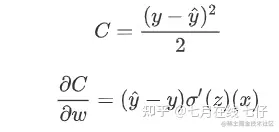Use the square loss function , You will find that the gradient update speed and sigmod The gradient of the function itself is very relevant .sigmod The gradient of a function in its domain is not greater than 0.25. This training will be very slow . Using cross entropy, this would not happen , Its derivative is a difference , If the error is large, the update is fast , If the error is small, update slowly , This is exactly what we want .

In the use of Sigmoid Function as the probability of a positive sample , At the same time, the square loss is taken as the loss function , At this time, the constructed loss function is nonconvex , Not easy to solve , It is easy to obtain its local optimal solution . If maximum likelihood is used , The objective function is the log likelihood function , The loss function is a higher-order continuously differentiable convex function with respect to unknown parameters , It is convenient to find the global optimal solution .（ About whether it is a convex function , From the definition of convex function , For unary functions , The second derivative is always nonnegative , For multivariate functions , Its Hessian matrix （Hessian A matrix is a square matrix composed of the second derivative of a multivariate function ） To judge . If Hessian A matrix is a semidefinite matrix ）

## 3.F1score Calculation formula .

To calculate F1score, First calculate Precision and Recall, The formula is as follows ：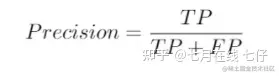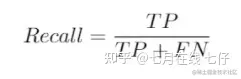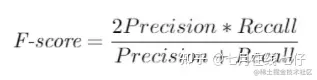## 4.FM vs SVM Comparison .

FM and SVM The biggest difference , The calculation method of weight in feature combination

• SVM The binary characteristic cross parameters are independent , and FM The binary characteristic cross parameter of is two k Dimension vector v_i,v_j , Cross parameters are not independent , But mutual influence
• FM Optimization learning can be carried out in the original form , And based on kernel The nonlinearity of SVM It usually needs to be done in dual form
• FM The model prediction is independent of the training samples , and SVM It is related to some training samples , Support vector .

FM The model has two advantages ：

In the case of high sparsity, the intersection between features can still be estimated , Moreover, it can be generalized to the learning of unobserved cross parameters and the time complexity of model prediction is linear

## 5. Randomness of random forest .

The randomness of random forest is reflected in that the training samples of each tree are random , The split attribute set of each node in the tree is also determined by random selection . With this 2 A random guarantee , Random forest will not produce over fitting phenomenon .

## 6. Programming questions ： jumping game (leetcode55)

Ideas ： Greedy Algorithm

Traverse each position in the array in turn , And maintain the farthest reachable location in real time rightMost, Note the current traversal position i Be within the farthest reachable position （ It's satisfaction i <= rightMost）, When rightMost Greater than or equal to At the last position of the array , It means you can reach , return True, Otherwise, return to False.

The code is as follows ：

``````class Solution:
def canJump(self, nums: List[int]) -> bool:
n = len(nums)
rightMost = 0
for i in range(n):
if i <= rightMost:
rightMost = max(rightMost, i + nums[i])
if rightMost >= n-1:
return True
return False
Copy code ``````

Time complexity ：O(n), among n For the size of the array . Just access nums Array again , common n A place .

Spatial complexity ：O(1), No additional space overhead .

Please bring the original link to reprint ,thank